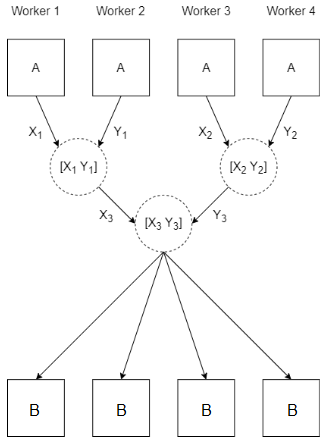# spmdCat

Concatenate arrays on `spmd` workers

## Syntax

``B = spmdCat(A)``
``B = spmdCat(A,dim)``
``B = spmdCat(A,dim,destination)``

## Description

example

````B = spmdCat(A)` horizontally concatenates the array `A` defined on each worker running an `spmd` block or communicating job.When you use `parfor`, `parfeval`, or `parfevalOnAll` to run code on a parallel pool, the workers are independent and do not communicate with each other. If you use `spmdCat` on these workers, the result is the same as using `spmdCat` on a client.You can use `spmdCat` only if `A` can be concatenated along the second dimension.This functions concatenates the arrays along the second dimension, then stores the result on all the workers. The function takes the value of `A` from each worker and concatenates the results in order of the worker index. To get the function of a worker, use the `spmdIndex` function. For example, `spmdCat(spmdIndex)` returns `1:spmdSize` , where `spmdSize` is the number of workers running the current spmd block or communicating job.If one worker is running the current `spmd` block, `B` is equal to `A`.```
````B = spmdCat(A,dim)` concatenates `A` along the dimension `dim`. You can use `spmdCat` only if `A` concatenates along the dimension `dim` when all the arrays have compatible sizes. The arrays have compatible sizes when the lengths of the dimensions match except for the operating dimension `dim`).```
````B = spmdCat(A,dim,destination)` concatenates `A` and stores the result on only one worker.```

## Examples

collapse all

This example shows how to use `spmdCat` to concatenate a scalar held on each worker in an `spmd` block.

Create a parallel pool with four workers.

`parpool(4);`

Create an `spmd` block. By default, each worker in the pool runs the `spmd` block. Store the value returned by `spmdIndex` on each worker as `A`, then use `spmdCat`to concatenate the values of `A` defined on each worker.

Unless you specify a destination, the `spmdCat` function stores the result of concatenation on every worker. On the client, the result is a `Composite` object. To get the result, index into the `Composite` object.

```spmd B = spmdCat(spmdIndex); end B{1}```
```ans = 1 2 3 4```

## Input Arguments

collapse all

Input array, specified as a scalar, vector, matrix, multidimensional array, table, timetable, or any MATLAB variable that supports concatenation.

Example: `A = magic(3)`

Dimension, specified as a positive integer. For example, if `A` is a 2-by-2 matrix on all workers, then `spmdCat(A,1)` concatenates A vertically and returns a 2N-by-2 matrix, where N is the number of workers running the current spmd block. Calling `spmdCat(A,2)` concatenates horizontally and returns a 2-by-2N matrix.

`dim` must be either `1` or `2` for table or timetable input.

Index of destination worker, specified as a positive integer. The value of this input must be less than or equal to the output of the `spmdSize` function in the current `spmd` block or communicating job.

When you specify this input, the function stores the value of `B` only on the worker with this index. The `B` output is still defined on all the workers that run `spmdCat`. On the worker with whose index is equal to `destination`, `B` is the result of the concatenation. On all the other workers, `B` is `[]`.

## Output Arguments

collapse all

Output array, returned as any MATLAB variable of the same type as `A`.

If you specify `destination`, `B` is the result of the concatenation on the worker whose index is equal to `destination`. On all the other workers, `B` is `[]`.

## Algorithms

This figure shows how the `spmdCat` function concatenates `A` with the value on other workers when you call `spmdCat(A)`.## Version History

Introduced in R2022b